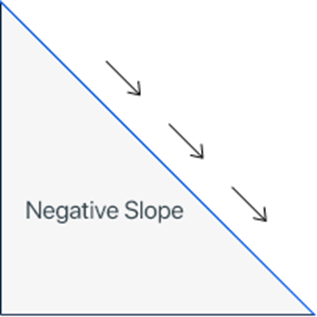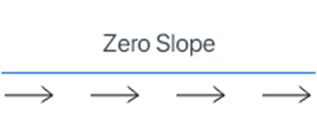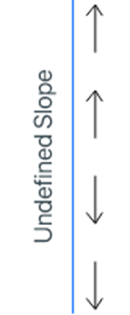## What is the Slope? Explained With ExampleThe slope is widely used in algebra for expressing various mathematical terms. Algebra is a branch of mathematics that deals with variables or symbols and uses arithmetic operations to determine the unknown quantity represented by these variables.

In this blog, we’ll discuss all the basics of the slope along with a lot of examples.

## What is the slope?

A slope is a measure of the steepness of the line. The slope remains unchanged anywhere on the line. A slope is the ratio of change in the value of y and the change in the values of x. In simple words, the ratio of rise and run is known as a slope.

Slope = m = rise/run

Slope = m = Δy/Δx = (y2-y1)/(x2-x1)

The value of the slope can be:
• Positive slope
• Negative slope
• Zero slope
• Undefined slope

The above-mentioned values of the slope are known as the types of the slope. Let us discuss them briefly.

### 1. Positive slope

A positive slope is a type of slope in which the object or a person moves from left to right to go upward.### 2. Negative slope

A negative slope is a type of slope in which the object or a person moves from left to right to go downward.### 3. Zero slope

A zero slope is a type of slope in which the object or a person moves from left to right on a straight path or line without moving upward or downward.### 4. Undefined slope

An undefined slope is a type of slope in which the object or a person does not move from left to right or right to left. In this type of slope, the object or a person has to face the vertical line to go up or down only.## Examples of the slope

The slope of the line can be calculated by using various methods like two points form or equation of the line. Following are a few solved examples of the slope.

### Example 1: By using the two points of the line

Find the slope of the line by using the points of the line (-1, 3) and (11, 33)?

Solution

Step 1:
Write the given points of the line.

x1 = -1, x2 = 11, y1 = 3, y2 = 33

Step 2: Write the general formula of the slope.

Slope = m = Δy/Δx = (y- y1)/(x- x1)

Step 3: Substitute the given points of the line in the formula of the slope.

Slope = m = Δy/Δx = (33 - 3) / (11 - (-1))
Slope = m = Δy/Δx = (33 - 3) / (11 + 1)
Slope = m = Δy/Δx = (30) / (12)
Slope = m = Δy/Δx = 30/12 = 5/2 = 2.5

Hence the slope of the line by using the given points (-1, 3) and (11, 33) is 2.5

### Example 2: By using the equation of the line

Find the slope of the line by using the given line equation.
12x + 16y + 8 = 0

Solution

Step 1: Take the given equation of the line and arrange it according to the equation of the slope-intercept for y = mx + b.

12x + 16y + 8 = 0
12x + 16y = -8
16y = - 12x -8
y = (- 12x -8)/16
y = -12x/16 – 8/16

Step 2: Simplify the fractions on the right side of the equation.

y = -3x/4 – 1/2
y = -0.75x – 0.5

Step 3: Compare the above equation with the equation of the slope-intercept form.

y = mx + b
y = -0.75x – 0.5

Hence, by comparing the terms we’ll get.

Slope of the line = m = -0.75
Y-intercept form = b = -0.5

## Summary

The slope of the line is widely used in algebra to find the equations of slope-intercept form, point-slope form, and the x & y intercept form. In this blog, we’ve discussed the slope, its formula, types, and examples.

## FAQs

Here are a few FAQs of the slope.

What is the slope of (0, 10) and (24, 6)?

The slope of (0, 10) and (24, 6) is -0.17

What is the slope of (0, 6) and (20, 14)?

The slope of (0, 6) and (20, 14) is 0.4

What is the slope of (1, 4) and (9, 4)?

The slope of (1, 4) and (9, 4) is 0

What is the slope of (-1, -14) and (19, 4)?

The slope of (-1. -14) and (19, 4) is 0.9